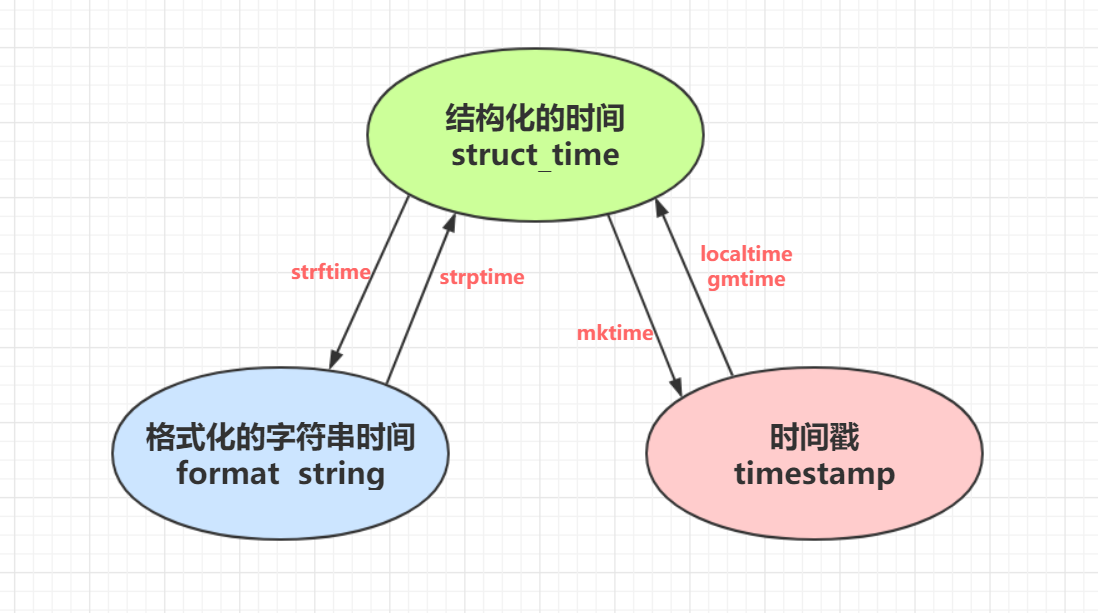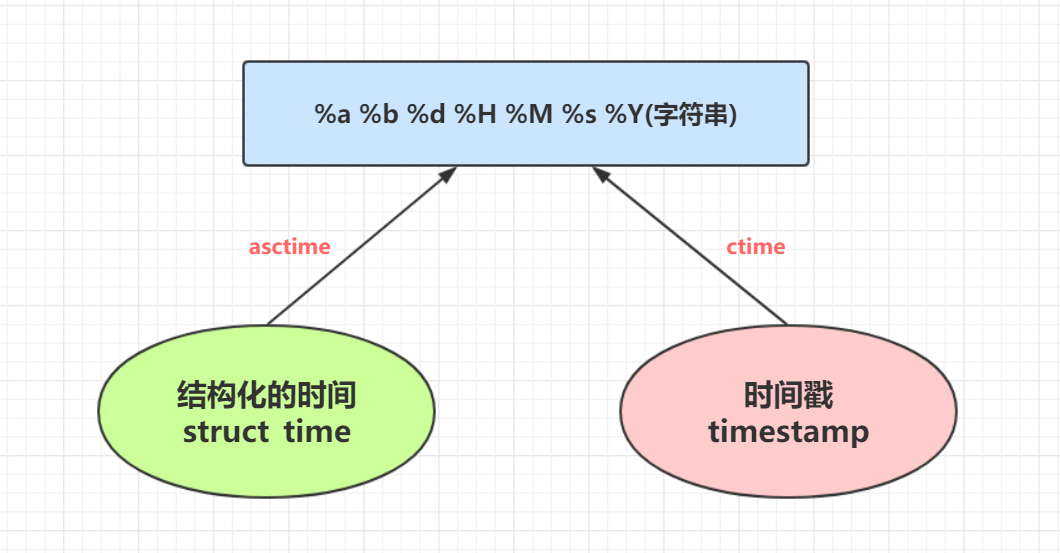# 44 time 与 datetime 模块

73次阅读

## 引入

### 1. 什么是 time 与 datetime 模块

• 它们是 Python 中与 时间处理 有关的 标准库模块

ps : Python 时间处理模块还有 calendar 模块

### 2.UTC 和 DST

• UTC（Coordinated Universal Time，世界协调时）格林威治天文时间，世界标准时间, 在中国 : `UTC+8`
• DST（Daylight Saving Time）夏令时

## 一. Python 中时间的三种表达方式

### 1. 时间戳 (timestamp)

• 通常来说，时间戳表示的是从 1970 年 1 月 1 日 00:00:00 开始 按秒计算 偏移量
• 返回时间戳方式的函数主要有 time()，clock()等, 运行 `print(type(time.time()))`, 返回的是float 类型
``````import time

print(time.time())        # 1608256754.2573004
print(type(time.time()))  # <class 'float'>
``````

### 2. 格式化的时间字符串 (Format String)

• 示例
``````import time

print(time.strftime("%Y-%m-%d %H:%M:%S %p"))  # 2020-12-18 10:25:16 AM
print(time.strftime("%Y-%m-%d %X"))  # 2020-12-18 10:25:16 AM
``````
• #### python 中时间日期格式化符号 :

%y 两位数的年份表示（00-99）
%Y 四位数的年份表示（000-9999）
%m 月份（01-12）
%d 月内中的一天（0-31）
%H 24 小时制小时数（0-23）
%I 12 小时制小时数（01-12）
%M 分钟数（00=59）
%S 秒（00-59）
%a 本地简化星期名称
%A 本地完整星期名称
%b 本地简化的月份名称
%B 本地完整的月份名称
%c 本地相应的日期表示和时间表示
%j 年内的一天（001-366）
%p 本地 A.M. 或 P.M. 的等价符
%U 一年中的星期数（00-53）星期天为星期的开始
%w 星期（0-6），星期天为星期的开始
%W 一年中的星期数（00-53）星期一为星期的开始
%x 本地相应的日期表示
%X 本地相应的时间表示
%Z 当前时区的名称
%% % 号本身

### 3. 结构化的时间 (struct_time) 元组

• struct_time 元组共有九个元素
• 返回 struct_time 的函数主要有 : localtime(), gmtime(), strptime()
``````import time

⛅localtime( )
print(time.localtime())        # 本地时区元组(struct_time)
''' 输出
time.struct_time(tm_year=2020, tm_mon=12, tm_mday=18, tm_hour=8, tm_min=45, tm_sec=9, tm_wday=4, tm_yday=353, tm_isdst=0)\
'''print(type(time.localtime()))  # <class'time.struct_time'>
print(time.localtime())     # 8

⛅gmtime( )
print(time.gmtime())           # UTC 时区元组(struct_time)
''' 输出
time.struct_time(tm_year=2020, tm_mon=12, tm_mday=18, tm_hour=0, tm_min=45, tm_sec=9, tm_wday=4, tm_yday=353, tm_isdst=0)
'''print(type(time.gmtime()))     # <class'time.struct_time'>
print(time.gmtime())        # 2020
``````
• #### 元組 (struct_time) 九个元素的含义 :

 索引 （Index） 属性 （Attribute） 值 （Values） 0 tm_year（ 年 ） 比如 2011 1 tm_mon（ 月 ） 1 - 12 2 tm_mday（ 日 ） 1 - 31 3 tm_hour（ 时 ） 0 - 23 4 tm_min（ 分 ） 0 - 59 5 tm_sec（ 秒 ） 0 - 59 6 tm_wday（weekday） 0 - 6（0 表示周日 ） 7 tm_yday（ 一年中的第几天 ） 1 - 366 8 tm_isdst（ 是否是夏令时 ） 默认为 -1

## 二. 时间格式转换关系 (需要掌握)

### 1. 转换图

• 计算机能认识的时间只能是 "时间戳" 格式
• 而人类能看懂的时间是 "格式化的时间字符串""结构化的时间", 于是就有了转换关系### 2. 格式转换操作 (需要掌握的操作)

• `格式化的字符串时间 ``时间戳 ` 之间的转换都是以 ` 结构化的时间 ` 作为 中转站 来进行操作的

• 🔰`结构化时间 ``时间戳 ` 之间的转化

• `time.mktime([结构化时间])` : "struct_time" 转换 "timestamp"
• `time.localtime([时间戳])` : "timestamp" 转换 "struct_time" 本地时区
• `time.gmtime([时间戳])` : "timestamp" 转换 "struct_time" UTC 时区
• `time.gmtime([time.time()])`
``````import time

⛅"struct_time" 转换 "timestamp"
print(time.mktime(time.localtime()))   # 1608259357.0
print(time.mktime(time.gmtime()))      # 1608259357.0

⛅"timestamp" 转换 "struct_time"
print(time.localtime(456465.4685))  # 返回的是 "struct_time" 本地时区元组
print(time.localtime(time.time()))  # 里面不填时间戳默认就是当前时间戳 "time.time()"

print(time.gmtime(456465.4685))     # 返回的是 "struct_time"UTC 时区元组
print(time.gmtime(time.time()))
``````
• 🔰`结构化时间 ``格式化字符串时间 ` 之间的转换
• `time.strftime([时间格式],[结构化时间])` : "struct_time" 转换 "format time"
• `time.strptime([格式化的字符串时间],[时间格式])` : "format time" 转换 "struct_time"
``````import time

⛅"struct_time" 转换 "format time"
print(time.strftime("%Y/%m/%d %X %p"))        # 2020/12/18 10:53:28 AM (%X 代表时分秒)
print(time.strftime("%Y-%m-%d %H:%M:%S %p"))  # 2020-12-18 10:53:28 AM

res = time.strftime("%Y-%m-%d %H:%M:%S %p",time.localtime())
print(res)  # 2020-12-18 10:58:22 AM (第二个参数填写的是 " 结构化时间 ", 默认就是当前时间的结构化时间)
res2 = time.strftime("%Y-%m-%d %H:%M:%S %p",time.gmtime(16545.5163))
print(res2) # 1970-01-01 04:35:45 AM

⛅"format time" 转换 "struct_time"
print(time.strptime('2020-12-18 10:58:22 AM','%Y-%m-%d %H:%M:%S %p'))
# 第一个参数是格式化字符串时间, 第二个参数是对应的格式, 必须一一对应, 不然报错, 得到的是 struct_time 元组
'''time.struct_time(tm_year=2020, tm_mon=12, tm_mday=18, tm_hour=10, tm_min=58, tm_sec=22, tm_wday=4, tm_yday=353, tm_isdst=-1)'''
print(time.strptime('1970-01-01 04:35:45 AM','%Y-%m-%d %H:%M:%S %p'))
'''time.struct_time(tm_year=1970, tm_mon=1, tm_mday=1, tm_hour=4, tm_min=35, tm_sec=45, tm_wday=3, tm_yday=1, tm_isdst=-1)'''
``````
• 🔰我们需要掌握的就是 ` 格式化的字符串时间 ``时间戳之间 ` 的转换
``````⛅"format time" 与 "timestamp" 转换
res = '2020-12-18 11:03:12 AM'        # 当前时间字符串
struct_time = time.strptime(res,'%Y-%m-%d %H:%M:%S %p')  # 先转成格式化字符, 格式必须一一对应
timestamp = time.mktime(struct_time)  # 再转成时间戳
print(timestamp)  # 1608260592.0

⛅时间加减, 以当前时间戳为起始来计算
res2 = timestamp + 24*3600  # 一天之后时间戳
print(res2)  # 1608346992.0
res3 = timestamp - 24*3600  # 一天之前时间戳
print(res3)  # 1608174192.0

⛅将加减之后的 " 时间戳 " 转换成 "format time"
struct_time = time.localtime(res2)  # 先转换成格式化时间
format_time = time.strftime("%Y-%m-%d %X %p",struct_time)  # 再转换成格式化时间字符串
print(format_time)  # 2020-12-19 11:03:12 AM (一天之后的时间)

struct_time = time.localtime(res3)
format_time = time.strftime("%Y-%m-%d %X %p",struct_time)
print(format_time)  # 2020-12-17 11:03:12 AM (一天之前的时间)
``````

## 三.time 模块其他常用方法

### 1.time.sleep(n)

• 推迟指定的时间 (单位秒) 之后继续运行, 我们通常戏称睡多少秒之后
``````import time

def sleep(n):
time.sleep(n)
print(f" 睡了 {n} 秒之后才打印的我 ")

sleep(3)  # 睡了 3 秒之后才打印的我
``````

ps : 做爬虫以及破解账号的时候, 程序需要模拟人类的行动来进行操作, 人类不可能有机器那么快的速度, 所以一般放一些 "sleep" 来模拟人类的慢动作, 以免被检测为机器而被掐断连接

ps2 : 推荐一篇博客 : 以故事话爬虫

## 四. 另一种时间格式转换方法 (只需了解)### 1.asctime()

• 把一个表示时间的元组或者 struct_time 表示为: "Sun Jun 20 23:21:05 1993" 这种形式
• 如果没有参数传入, 则默认传入 `time.localtime()`
``````import time

print(time.asctime())  # Fri Dec 18 18:22:04 2020
print(time.asctime(time.localtime()))  # Fri Dec 18 18:22:04 2020
``````

### 2.ctime()

• 把一个时间戳(按秒计算的浮点数) 转换为: "Sun Jun 20 23:21:05 1993" 这种形式
• 如果没有传入参数, 则默认传入`time.time()`
• 作用等同于 : `time.asctime(time.localtime(n))`, "n" 表示秒
``````import time

print(time.ctime())  # Fri Dec 18 18:26:37 2020
print(time.ctime(time.time()))  # Fri Dec 18 18:26:37 2020
print(time.ctime(5456161.56456))  # Thu Mar  5 11:36:01 1970
``````

ps : 在 Linux 系统上这种格式比较常见, 等同于: `time.strftime('%b %a %d %X %Y')`

## 五.datetime 模块

• 时间加减运算

### 2.time 模块与 datetime 模块的不同点

• datetime 可以在原本的时间结构上参与时间的运算
• time 只有在时间戳的基础上才能进行运算, 运算之后在进行格式之间的转换

### 3. 常用功能介绍

• `datetime.now()` : 统计当前时间
``````import datetime

print(datetime.datetime.now())    # 2020-12-18 20:56:58.262592 (本地时间)
print(datetime.datetime.utcnow()) # 2020-12-18 12:56:58.262592 (世界标准时间)
``````
• `date.fromtimestamp()` : 将时间戳直接转成格式化字符串的時間
``````import datetime

print(datetime.date.fromtimestamp(5465564.564))  # 1970-03-05
print(datetime.date.fromtimestamp(time.time()))  # 2020-12-18
``````
• `timedelta()` : 统计时间间隔与时间加减运算 (没有年份单位的计算)
``````
import datetime

print(datetime.datetime.now() + datetime.timedelta(-2))  # 两天前, 不写关键字默认 "day"
print(datetime.datetime.now() + datetime.timedelta(days=-2))     # 两天前
print(datetime.datetime.now() + datetime.timedelta(weeks=3))     # 三星期后
print(datetime.datetime.now() + datetime.timedelta(hours=-3))    # 三小时前
print(datetime.datetime.now() + datetime.timedelta(seconds=10))  # 十秒后
print(datetime.datetime.now() + datetime.timedelta(minutes=10))  # 十分钟后
print(datetime.datetime.now() + datetime.timedelta(minutes=10, seconds=30)) # 十分钟 30 秒后
``````
• `replace()`: 时间替换
``````import datetime

now_time = datetime.datetime.now()
print(now_time)                                      # 2020-12-18 20:53:34.602813

print(now_time.replace(minute=00,hour=00,second=00)) # 2020-12-18 00:00:00.267086
print(now_time.replace(day=11,month=11,year=1111))   # 1111-11-11 20:53:34.602813
``````